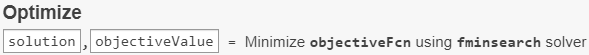Main Content

## Optimize Live Editor Task

### What Is the Optimize Live Editor Task?

The Optimize Live Editor task provides a visual interface for the `fminbnd`, `fminsearch`, `fzero`, and `lsqnonneg` solvers. To start the task, click the button. Then click the Insert tab and select Task > Optimize.### Minimize a Nonlinear Function of Several Variables

This example shows how to minimize the function $f\left(x,y\right)=100{\left(y-{x}^{2}\right)}^{2}+{\left(a-x\right)}^{2}$ where the variable a = π using the Optimize Live Editor task.

For a video describing a similar optimization problem, see How to Use the Optimize Live Editor Task.

1. On the Home tab, in the File section, click the button.

2. Insert an Optimize Live Editor task. Click the Insert tab and then, in the Code section, select Task > Optimize.3. For use in entering problem data, click the button. New sections appear above and below the task.

4. In the section above the Optimize task, enter the following code.

```a = pi; x0 = [-1 2];```
5. To place these variables into the workspace, press Ctrl + Enter.

6. In the Specify problem type section of the task, click the button and the button. The task shows that the recommended solver is `fminsearch`.

Note

If you have Optimization Toolbox™, your recommended solver at this point is different. Choose `fminsearch` to proceed with the example.

7. In the Select problem data section, select Objective function > Local function and then click the button. A function script appears in a new section below the task. Edit the resulting code to contain the following uncommented lines.

```function f = objectiveFcn(optimInput,a) x = optimInput(1); y = optimInput(2); f = 100*(y - x^2)^2 + (a - x)^2; end```
8. In the Select problem data section, select `objectiveFcn` as the local function.

9. In the Select problem data section, under Function inputs, select Optimization input > optimInput and Fixed input: a > a.10. Select Initial point (x0) > x0.

11. In the Display progress section, select Objective value for the plot.

12. To run the solver, click the options button at the top right of the task window, and select Run Section.The following plot appears.13. To view the solution point, look at the top of the Optimize task.The `solution` and `objectiveValue` variables are returned to the workspace. To view their values, insert a section break below the task and enter this code.

```disp(solution) disp(objectiveValue)```
14. Run the section by pressing Ctrl+Enter.

`disp(solution)`
` 3.1416 9.8696`
`disp(objectiveValue)`
` 3.9946e-11`

### Solve a Scalar Equation

This example shows how to use the Optimize Live Editor task to find the point x where cos(x) = x.

1. On the Home tab, in the File section, click the button. Enter these lines of code in the live script.

```fun = @(x)cos(x) - x; x0 = 0;```

The first line defines the anonymous function `fun`, which takes the value 0 at the point x where cos(x) = x. The second line defines the initial point `x0` = 0, where `fzero` begins its search for a solution.

2. Put these variables in the MATLAB® workspace by pressing Ctrl+Enter.

3. Insert an Optimize Live Editor task. Click the Insert tab and then, in the Code section, select Task > Optimize.

4. In the Specify problem type section of the task, select Solver > fzero.

5. In the Select problem data section, select Objective function > Function handle and then select `fun`. Select Initial point (x0) > x0.6. In the Display progress section, select for the plot.7. Run the solver by pressing Ctrl+Enter.8. To see the solution value, insert a new section below the task by clicking the button on the Insert tab. In the new section, enter `solution` and press Ctrl+Enter.

`solution`
`solution = 0.7391`

Download ebook